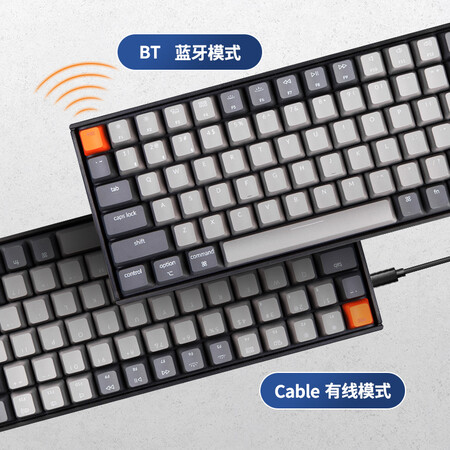# 香蕉吧啦-JavaScript怪异行为集合（一）[广告]京东京造 K2蓝牙双模机械键盘 背光84键有线/蓝牙无线双模

### 1 Not a Number是一个数字

``typeof NaN; // 'number'``

### 2   + ‘a’ = ?

``('b'+'a'+ +'a' +'a').toLowerCase();``### 3 最大值比最小值小

``````Math.min(1, 4, 7, 2); // 1
Math.max(1, 4, 7, 2); // 7``````

``````Math.min(); // Infinity
Math.max(); // -Infinity
Math.min() > Math.max(); // true``````-Infinity is the initial comparant because almost every other value is bigger, that’s why when no arguments are given, -Infinity is returned.

-Infinity是初始比较值，因为几乎所有其他的值都比较大，这就是为什么当没有给出参数时，-Infinity会被返回。

### 4 parseInt(null,24)

• string：被解析的字符串（前后空格会被忽略，若不是字符串，则转换成字符串）
• radix：指定 `string（第一个参数）`的基数（2~36，即 `2 进制` ~ `36进制`），若不传或传 `0`，则 `string（第一个参数）`以 `10 进制` 来解析

Base-24 Conversion Tool### 5 那么 parseInt(NaN,24) 等于多少？

NaN 与 Null 第1个字符都是N,那应该是也是23吧。

`23 * 242 + 10 * 241 + 23 * 240  = 13511`

### 参考资料